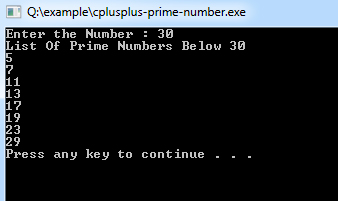# C++ Program to Find Prime Number

A prime number (or a prime) is a natural number greater than 1 that has no positive divisors other than 1 and itself.

Example:
``````#include <iostream>
#include <math.h> //sqrt function used from this library

using namespace std;

int main(){
// Variable Declaration
int n;

// Get Input Value
cout<<"Enter the Number : ";
cin>>n;

cout <<"List Of Prime Numbers Below "<<n<<endl;

//for Loop Block For Find Prime Number

for (int i=2; i<n; i++)
for (int j=2; j*j<=i; j++)
{
if (i % j == 0)
break;
else if (j+1 > sqrt(i)) {
cout << i << endl;
}
}
return 0;
}``````
Program Output:Explanation:

This program is used to generate all the prime numbers from 1 till the number given by the user. So, first of all, you have to include the iostream header file using the "include" preceding by # which tells that hat the header file needs to be process before compilation, hence named preprocessor directive. Now, for removing naming conflict you can use namespace statement within a program.

Now you have to declare the main() function and inside that main() definition, declare a variable of type integer with name 'n'. Then using cout<<""; prints the string "Enter the Number : ". The cin statement is used to read the value from the keyboard. Then a for loop will be implemented which will count from 2 till n-1. Another nested loop is implemented which goes from j=2 till j*j<=i.

Then a condition is checked when i % j == 0, break statement gets executed else (j+1 > sqrt(i)) And using cout prints the value of i. The system("PAUSE") is used to pause the console of the system. And finally the return 0; statement is used to return an integer type value back to main().